• 谭浩强 C语言程序设计第五版 第4章课后答案 3.求两个正整数m和n，求其最大公约数和最小公倍数。 #include<stdio.h> void main() { int m, n, t, i, a = 1; scanf("%d%d", &m, &n); if (m < n) ...
谭浩强 C程序设计第五版 第五版课后答案
点我看视频讲解+可运行源码记得一键三连哦
c程序设计
第一章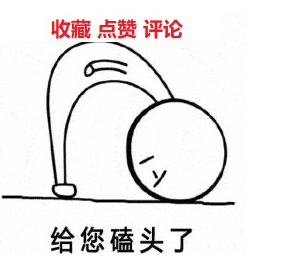1.什么是程序?什么是程序设计?

程序：就是一组能识别和执行的指令，每一条指令使计算机执行特定的操作

程序设计：是指从确定任务到得到结果、写出文档的全过程

2.为什么需要计算机语言?高级语言有哪些特点?

为什么需要计算机语言：计算机语言解决了人和计算机交流是的语言问题，使得计算机和人都能识别

高级语言有哪些特点：
2.1 高级语言的数据结构要比汇编和机器语言丰富；
2.2 高级语言与具体机器结构的关联没有汇编以及机器语言密切；
2.3 高级语言更接近自然语言更容易掌握；
2.4 高级语言编写的程序要经过编译或解释计算机才能执行;

3.正确理解以下名词及其含义:
(1)源程序,目标程序,可执行程序。

​源程序：指未编译的按照一定的程序设计语言规范书写的文本文件，是一系列人类可读的计算机语言指令

目标程序：为源程序经编译可直接被计算机运行的机器码集合，在计算机文件上以.obj作扩展名

可执行程序：将所有编译后得到的目标模块连接装配起来，在与函数库相连接成为一个整体，生成一个可供计算机执行的目标程序，成为可执行程序

(2)程序编辑,程序编译,程序连接。
程序编辑：上机输入或者编辑源程序。
程序编译：

先用C提供的“预处理器”，对程序中的预处理指令进行编译预处理
对源程序进行语法检查， 判断是否有语法错误，直到没有语法错误未知
编译程序自动把源程序转换为二进制形式的目标程序

程序连接：将所有编译后得到的目标模块连接装配起来，在与函数库相连接成为一个整体的过程称之为程序连接
(3)程序,程序模块,程序文件。

程序：一组计算机能识别和执行的指令，运行于电子计算机上，满足人们某种需求的信息化工具

程序模块：可由汇编程序、编译程序、装入程序或翻译程序作为一个整体来处理的一级独立的、可识别的程序指令

程序文件：程序的文件称为程序文件，程序文件存储的是程序，包括源程序和可执行程序

(4)函数,主函数，被调用函数,库函数。

函数：将一段经常需要使用的代码封装起来，在需要使用时可以直接调用，来完成一定功能

主函数：又称main函数，是程序执行的起点

被调用函数：由一个函数调用另一个函数，则称第二个函数为被调用函数

库函数：一般是指编译器提供的可在c源程序中调用的函数。可分为两类，一类是c语言标准规定的库函数，一类是

​		编译器特定的库函数
(5)程序调试,程序测试。

程序调试：是将编制的程序投入实际运行前，用手工或编译程序等方法进行测试，修正语法错误和逻辑错误的过程

程序测试：是指对一个完成了全部或部分功能、模块的计算机程序在正式使用前的检测，以确保该程序能按预定的方式正确地运行

4.编写一个C程序,运行时输出
Hello World!
这个程序是一些国外C教材中作为第一个程序例子介绍的，一般称为Hello程序。
代码示例：
#include <stdio.h>

int main()
{
printf("%s\n", "Hello World!");
return 0;
}

运行截图：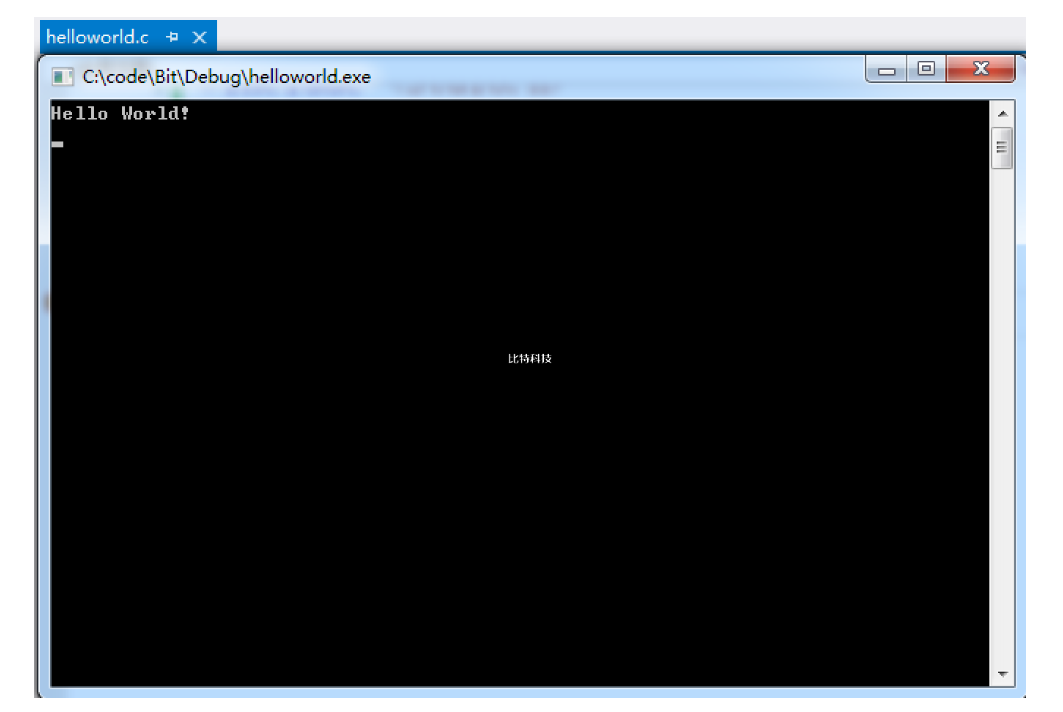5.编写一个C程序,运行时输出以下图形:
****
****
​    ****
​      ****
代码示例：
#include <stdio.h>

int main()
{
for (int i = 0; i < 4; i++)
{
for (int j = 0; j < i; j++)
{
printf("%s", "  ");
}
printf("%s\n", "*****");
}
return 0;
}

运行截图：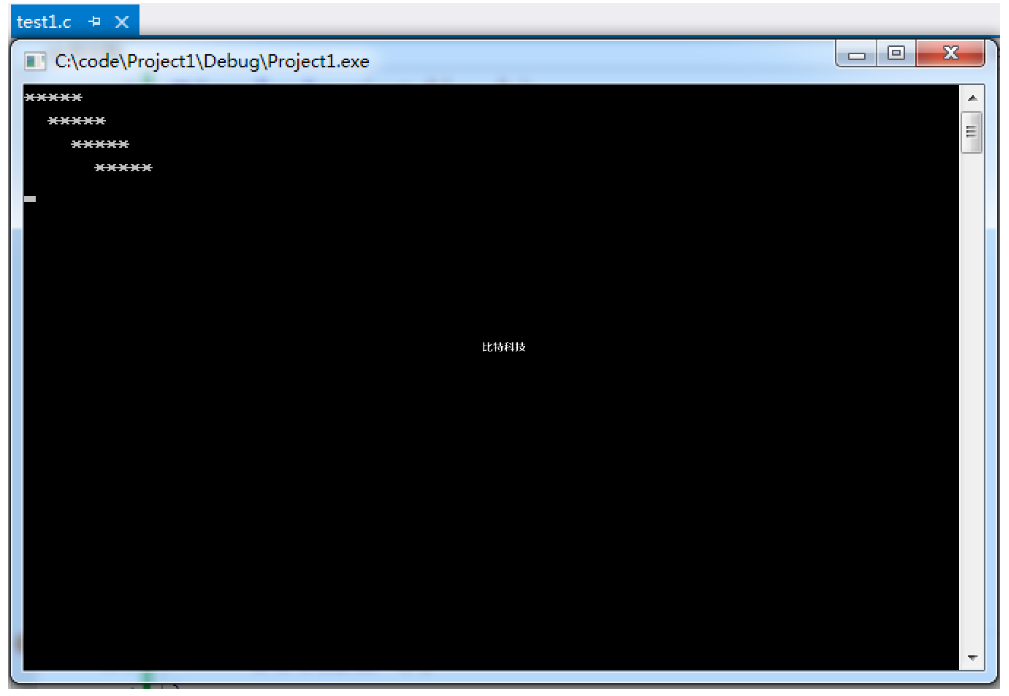6.编写一个C程序,运行时输人a,b,c三个值,输出其中值最大者。
代码示例：
#include<stdio.h>

int main()
{
int a, b, c, max;
printf("请输入三个数：\n");

scanf("%d%d%d", &a, &b, &c);
if (a > b)
{
max = a;
}
else
{
max = b;
}

if (c > max)
{
max = c;
}
printf("三个数中最大的数为：%d", max);
return 0;

}

运行截图：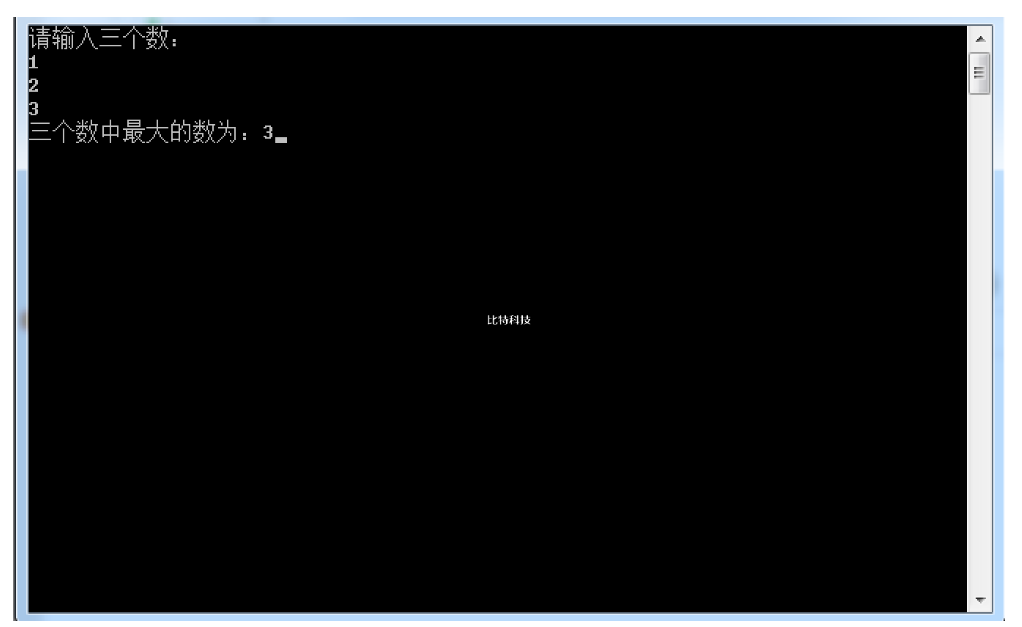7.看懂《C程序设计(第五版)学习辅导》第16章中介绍的用Visual Studio 2010对C程序进行编辑、编译、连接和运行的方法,并进行以下操作:
(1)建立一个新项目,定名为project1.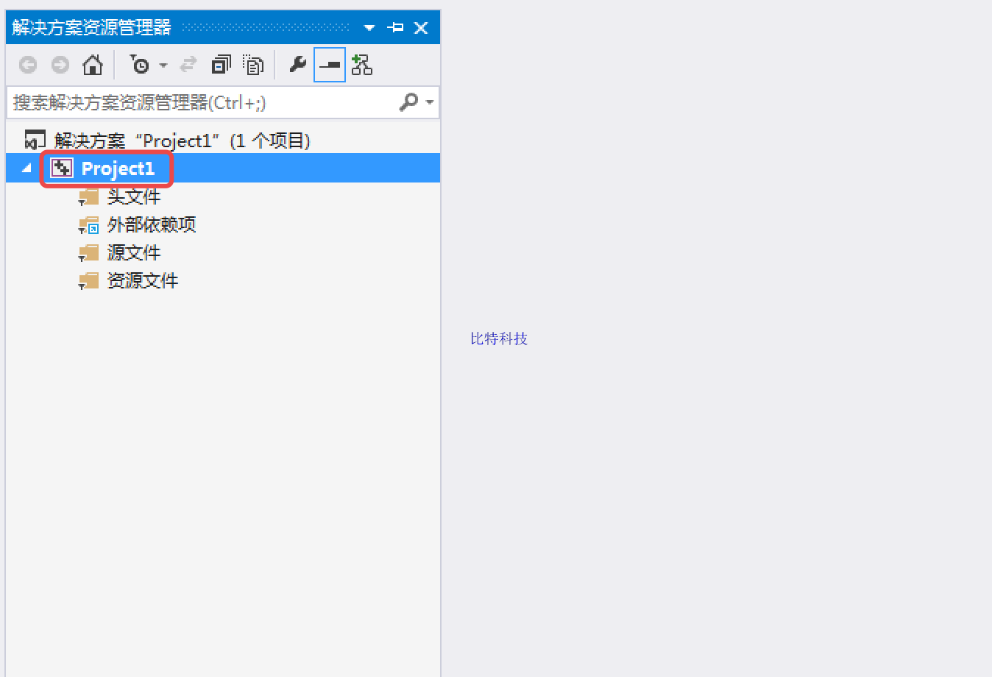(2)建立一个新文件,定名为test1。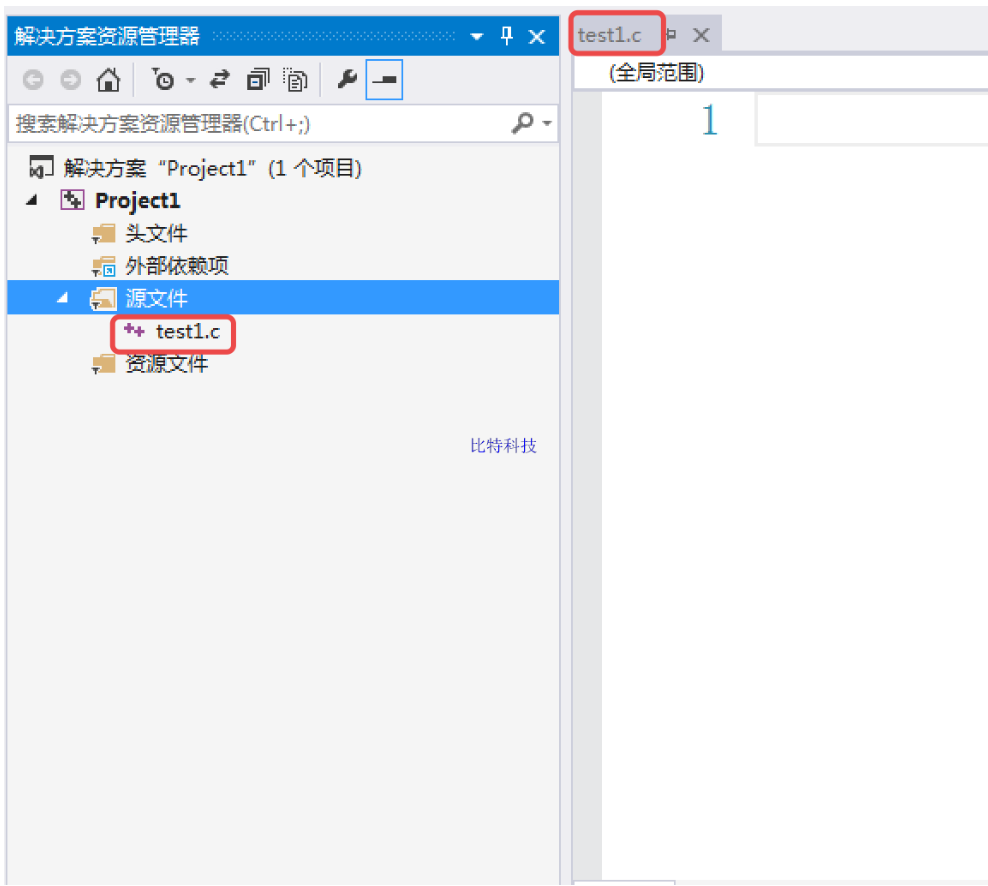(3)向test1 文件输人源程序(此源程序为读者自己编写的程序)。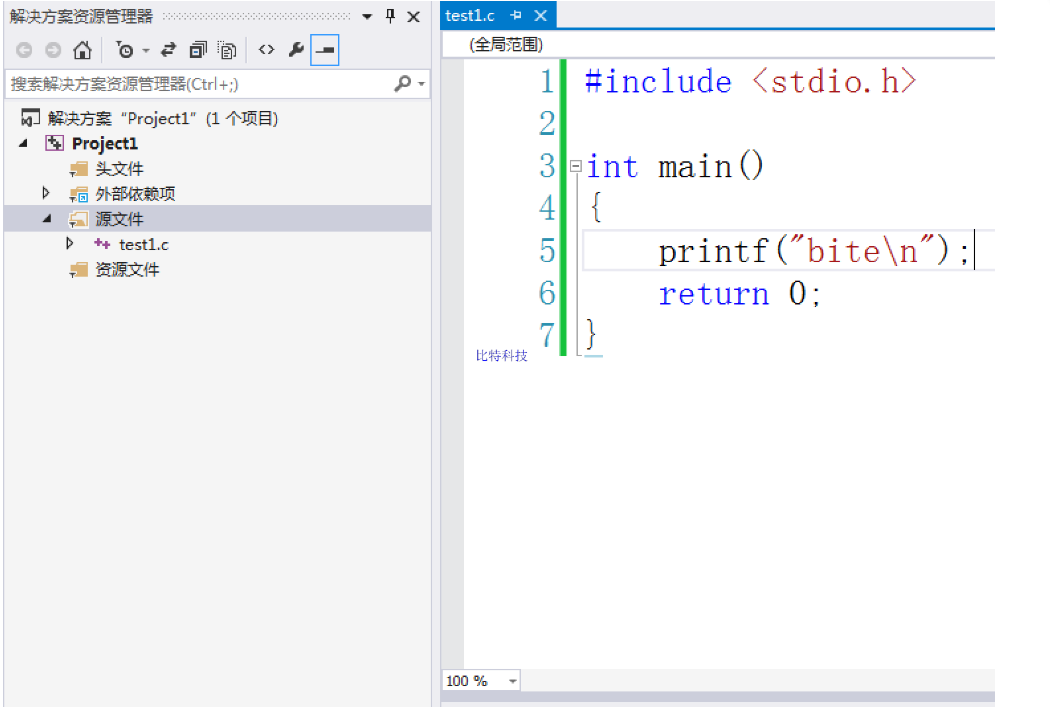(4)编译该源程序,如发现程序有错，请修改之,直到不出现“编译出错”为止。
(5)连接并运行, 得到结果。分析结果。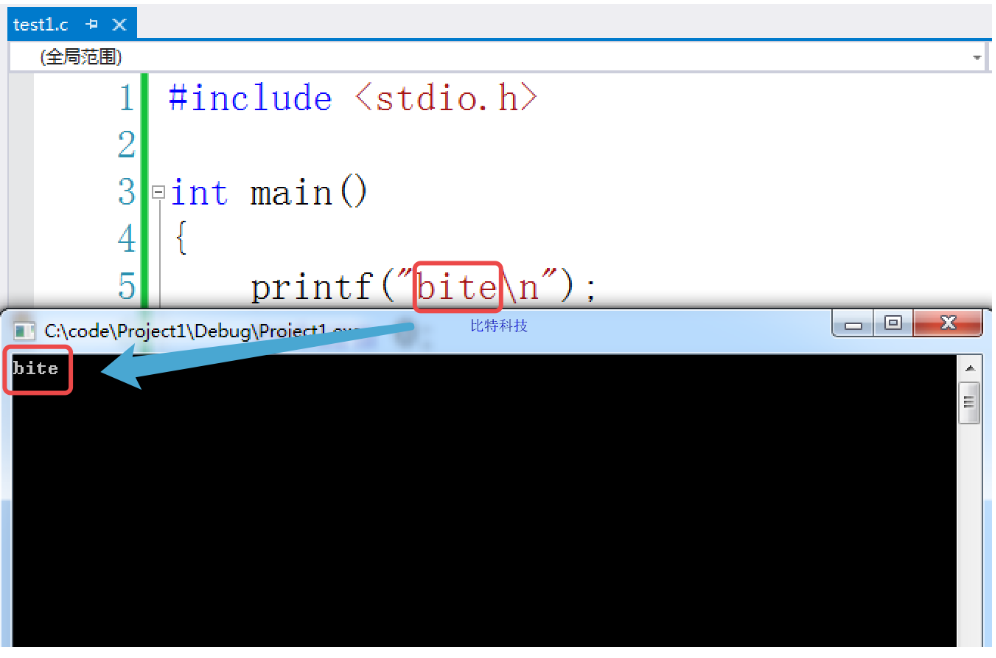点我看视频讲解+可运行源码记得一键三连哦
更多相关文章
C程序设计第五版谭浩强课后习题答案 第一章
C程序设计第五版谭浩强课后习题答案 第二章
C程序设计第五版谭浩强课后习题答案 第三章
C程序序设计第五版谭浩强课后习题答案 第四章
C语言程序设计第五版谭浩强课后习题答案 第五章
C语言程序设计第五版谭浩强课后习题答案 第六章
C语言程序设计第五版谭浩强课后习题答案 第七章
C语言程序设计第五版谭浩强课后习题答案 第八章
C语言程序设计第五版谭浩强课后习题答案 第九章
C语言程序设计第五版谭浩强课后习题答案 第十章


展开全文• ## C语言程序设计 课程设计报告

千次阅读 多人点赞 2020-06-17 17:39:14
C语言程序设计课程设计报告 一、 系统简介 学生成绩管理系统简介：一个简易的学生成绩管理系统，它包括并可以实现：“录入并保存学生信息、打印学生信息、读取学生信息、查找学生信息、删除学生信息、修改学生信息、...
C语言程序设计课程设计报告
一、	系统简介
学生成绩管理系统简介：一个简易的学生成绩管理系统，它包括并可以实现：“录入并保存学生信息、打印学生信息、读取学生信息、查找学生信息、删除学生信息、修改学生信息、排序学生信息、退出系统 ”等功能，此系统需要使用者准确录入学生信息，已达到高效的工作需求。
二、	设计要求
本次课程设计要求用C语言编程实现一个小型的学生成绩管理系统，每个结构的数据可以包括学号、姓名、平时成绩、作业成绩和期末成绩等。
程序采用简单菜单驱动方式，屏幕上显示菜单如下：
1—	录入并保存学生信息
2—	打印学生信息
3—	读取学生信息
4—	查找学生信息显示
5—	删除学生信息
6—	修改学生信息
7—	排序学生信息
8—	退出系统
由两个同学组成一组分工合作完成本次课程设计。本人的主要工作包括如下内容：
1-录入并保存学生信息
2-读取学生信息
3-查找学生信息显示
4-删除学生信息
三、	程序流程图
（此部分要求画出系统的主要功能图，自己所负责具体功能模块的流程图）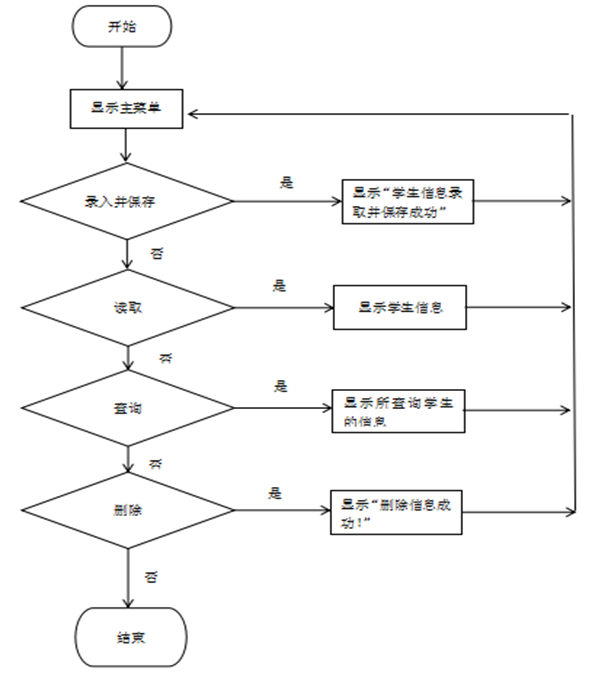四、	程序源代码
（要求程序中要有注释，培养规范化编程习惯）
部分代码参考
	#include<stdio.h>
#include<conio.h>//getch的头文件
#include<stdlib.h>//system的头文件
#include<string.h>
//定义一个学生
typedef struct TagStudent
{
int Num;//学号
char Name;//姓名
float Ascore;//平时成绩
float Hscore;//作业成绩
float Tscore;//考试成绩
float total;//总成绩
struct TagStudent *next;//next指针变量 ，指向结构体变量
}Student;

	Student *head=NULL;
void InputStudent();
void PrintStudent();
void SearchStudent();
void DeleteStudent();
void ChangStudent();
void SortStudent();
void sortStrArr(Student *list, int n);

int main()
{
while(1)
{
//	读取一个字符
char ch=getch();
//打印菜单
if (ch!= '2')
{
system("cls");

}
switch(ch)
{
case '1'://录入学生信息
InputStudent();
break;
case '2'://打印学生信息
PrintStudent();
break;
case '3'://浏览学生信息
break;
case '4'://查找学生信息
SearchStudent();
break;
case '5'://删除学生信息
DeleteStudent();
break;
case '6'://修改学生信息
ChangStudent();
break;
case '7'://排序学生信息
SortStudent();
break;
case '0'://退出系统
printf("欢迎下次使用.");
return 0;
break;
}
}
return 0;
}

{

printf("========欢迎使用学生成绩管理系统=======\n");//\t表示制表符
printf("***************************************\n");
printf("*\t    请选择功能列表            *\n");
printf("***************************************\n");
printf("*\t    1.录入并保存学生信息      *\n");
printf("*\t    2.打印学生信息            *\n");
printf("*\t    3.读取学生信息            *\n");
printf("*\t    4.查找学生信息            *\n");
printf("*\t    5.删除学生信息            *\n");
printf("*\t    6.修改学生信息            *\n");
printf("*\t    7.排序学生信息            *\n");
printf("*\t    0.退出系统                *\n");
printf("***************************************\n");

}

//创建一个保存链表

	Student *savestudent(Student *p)
{
FILE *fp=fopen("E:\\student.txt","w");
if(fp==NULL)
{
printf("打开文件失败\n");
return NULL;
}
//写入数据
{
}
fclose(fp);
return p;
}

//录入学生信息

void InputStudent()
{

//找到尾节点
{
p=p->next;
}
//定一个新节点
Student *NewNode=(Student*)malloc(sizeof(Student));
NewNode->next=NULL;

{
}
else{
p->next=NewNode;
}
printf("\n请输入学生信息\n ");
//输入新的学元数据
printf("         学号:");
scanf("%d", &NewNode->Num);
printf("         姓名:");
scanf("%s", NewNode->Name);
printf("     平时成绩:");
scanf("%f", &NewNode->Ascore);
printf("     作业成绩:");
scanf("%f", &NewNode->Hscore);
printf("     考试成绩:");
scanf("%f", &NewNode->Tscore);
FILE *fp=fopen("E:\\student.txt","a");
if(fp==NULL)
{
printf("打开文件失败\n");
return ;
}
//写入数据
while(p!=NULL)
{
fprintf(fp,"%d %s %.2f  %.2f  %.2f\n",p->Num,
p->Name,
p->Ascore,
p->Hscore,
p->Tscore);
p=p->next;
}
fclose(fp);
printf("学生信息录取并保存成功!\n");
}

// 创建生成链表并返回
Student *createLine(FILE *fp)
{
p1 = p2 = (Student*) malloc(sizeof(Student));//开辟一个新单元

while(!feof(fp))//feof(fp)文件未结束输出0
{
fscanf(fp,"%d%s%f%f%f\n",&p1->Num,
p1->Name,
&p1->Ascore,
&p1->Hscore,
&p1->Tscore);
p2 = p1;
p1 = (Student*) malloc(sizeof(Student));
p2->next = p1;

}
else p2->next = NULL;
}

// 从文件读取数据并生成返回链表 ，从文件中获取数据生成链表并返回链表的头指针

	Student *readFiles()
{
FILE *fp = fopen("E:\\student.txt", "r");
if(fp == NULL)
{
printf("打开文件失败!");
return NULL;
}
fclose(fp);
}

//读取学生信息
{ //打开文件

printf("学号\t姓名\t平时成绩\t作业成绩\t考试成绩\n");
while(p!=NULL)
{
printf("%d   \t%s    \t%.2f      \t%.2f      \t%.2f      \n",
p->Num,
p->Name,
p->Ascore,
p->Hscore,
p->Tscore);
p=p->next;
}
}
//查找学生信息
void SearchStudent()
{
char searchname;//空的字符数组；
printf("\n请输入你要查找的学生名字:");
scanf("%s",searchname);
while(strcmp(p->Name,searchname)!=0)
{
if(p->next==NULL)
{
printf("没有此学生的信息\n");
return ;
}
p=p->next;
}
printf(" 学号：\t姓名：\t平时成绩：\t作业成绩：\t考试成绩：\n");
printf("  %d   \t%s       %.2f          %.2f           %.2f      \n",
p->Num,
p->Name,
p->Ascore,
p->Hscore,
p->Tscore);
}

五、	运行结果及分析
（要求有程序运行结果截图和相应的分析）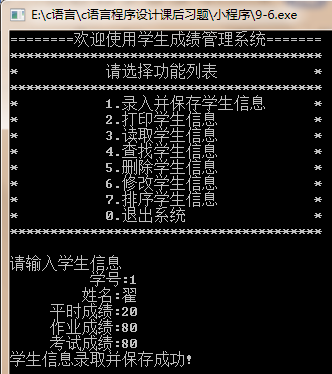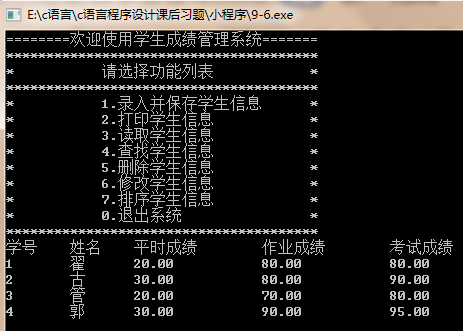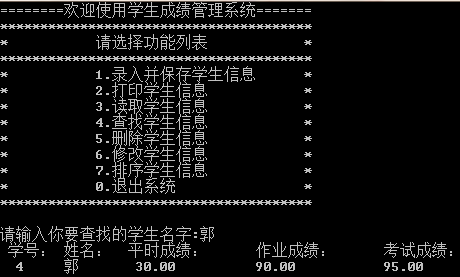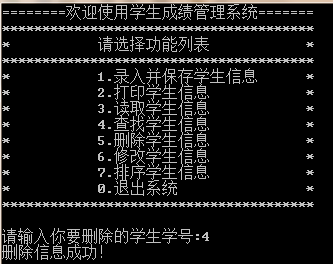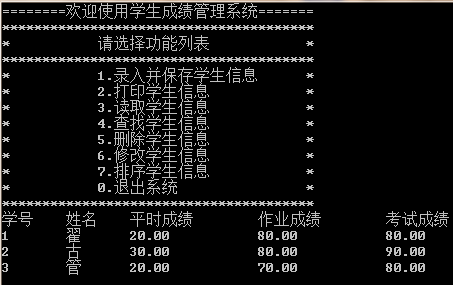六、	设计总结
（详述本次调试程序所取得的经验。如果程序未能通过，应分析其原因。）
学生成绩管理系统是一个十分强大的管理系统，起初刚开始做课程设计时,以为这个课设不会有多难,不过是把多个函数组合起来就好了,但是,经过这两星期的编程,发现要完整把程序按照要求编出来是一件很不容易的事情。在学习中,也对C语言有更加的了解。在整个编程过程中,可以说遇到了很多难题,在书和同学的
帮助下最后解决了这些问题，,我觉编写程序需要很大的耐心,当然细心也很需要，在编程的过程中有很多错误都是自己的粗心造成的,。这次的课程设计也是一次很好的对自我的检查，使我受益匪浅！
参考资料

C程序设计（第四版），谭浩强，清华大学出版社，2010年
C程序设计（第四版）学习辅导，谭浩强，清华大学出版社，2010年


展开全文编程语言
• C语言程序设计实习报告 简介 语言实践心得体会范文在科技高度发展的今天，计算机在人们之中的作用越来越突出。而c语言作为一种计算机的语言，我们学习它，有助于我们更好的了解计算机，与计算机进行交流，因此，...
C语言程序设计实习报告
简介
语言实践心得体会范文在科技高度发展的今天，计算机在人们之中的作用越来越突出。而c语言作为一种计算机的语言，我们学习它，有助于我们更好的了解计算机，与计算机进行交流，因此，c语言的学习对我们尤其重要。 在这个星期里，我们专业的学生在专业老师的带领下进行了c语言程序实践学习。在这之前，我们已经对c语言这门课程学习了一个学期，对其有了一定的了解，但是也仅仅是停留在了解的范围，对里面的好多东西还是很陌生，更多的在运用起来的时候还是感到很棘手，毕竟，万事开头难嘛。 由于时间的关系，我们的这次实践课程老师并没有给我们详细的介绍，只是给我们简单的介绍了几个比较重要的实际操作。包括了程序模块处理。简单界面程序。高级界面程序。程序的添加修改。用程序做一元线性回归处理以及用c语言程序来画粒度分布图等这几样比较重要的时间操作。 上机实验是学习程序设计语言必不可少的实践环节，特别是c语言灵活、简洁，更需要通过编程的实践来真正掌握它。对于程序设计语言的学习目的，可以概括为学习语法规定、掌握程序设计方法、提高程序开发能力，这些都必须通过充分的实际上机操作才能完成。学习c程序设计语言除了课堂讲授以外，必须保证有不少于课堂讲授学时的上机时间。因为学时所限，课程不能安排过多的统一上机实验，所以希望学生有效地利用课程上机实验的机会，尽快掌握用c语言开发程序的能力，为今后的继续学习打下一个良好的基础。为此，我们结合课堂讲授的内容和进度，安排了12次上机实验。课程上机实验的目的，不仅仅是验证教材和讲课的内容、检查自己所编的程序是否正确，课程安排的上机实验的目的可以概括为如下几个方面： 1．加深对课堂讲授内容的理解课堂上要讲授许多关于c语言的语法规则，听起来十分枯燥无味，也不容易记住，死记硬背是不可取的。然而要使用c语言这个工具解决实际问题，又必须掌握它。通过多次上机练习，对于语法知识有了感性的认识，加深对它的理解，在理解的基础上就会自然而然地掌握c语言的语法规定。对于一些内容自己认为在课堂上听懂了，但上机实践中会发现原来理解的偏差，这是由于大部分学生是初次接触程序设计，缺乏程序设计的实践所致。 学习c语言不能停留在学习它的语法规则，而是利用学到的知识编写c语言程序，解决实际问题。即把c语言作为工具，描述解决实际问题的步骤，由计算机帮助我们解题。只有通过上机才能检验自己是否掌握c语言、自己编写的程序是否能够正确地解题。 通过上机实验来验证自己编制的程序是否正确，恐怕是大多数同学在完成老师作业时的心态。但是在程序设计领域里这是一定要克服的传统的、错误的想法。因为在这种思想支配下，可能你会想办法去掩盖程序中的错误，而不是尽可能多地发现程序中存在的问题。自己编好程序上机调试运行时，可能有很多你想不到的情况发生，通过解决这些问题，可以逐步提高自己对c语言的理解和程序开发能力。
基本步骤
步骤2.打开Visual C++软件新建文件，点击文件-新建-在弹出的窗口中点击新建，选择C++Source File建立源文件，选择C++ Source，一般都是建立这种文件的（适用在当文件中适用）如果要建立头文件的话，选择C/C++ Header File，（适用在多文件工程中使用）命名，文件名称，点击确定，之后在右边的文件名出填写文件的名词，在位置处填写一个路径，这样编写程序后会在相应的位置声称源代码，点击确定，会弹出一个窗口，在窗口中即可编译程序。
1.C语言的概述
代码展示
#include <stido.h>
#include <math.h>
int main(void)
{
int a = 1;
int b = 2;
int c = 3;

float delta;
float x1;
float x2;

delta = b*b - 4*a*c;

if (delta > 0)
{
x1 = (-b + sqrt(delta)) / (2 * a);
x2= (-b - sqrt(delta)) / (2 * a);

printf("该一元二次方程有两个解，x1=%f,x2=%f\n", x1, x2);
}
else if （delta = 0）
{
x1 = -b / (2 * a);
x2 = x1;
printf("该一元二次方程有唯一一个解，x1=x2=%f\n", x1);
}

else
{
printf("无解\n");
}
return 0;
}
程序截图2.算法
代码展示
# include <stdio.h>

int main(void)
{
int i,j;
for(i = 100;i <= 300;i++)
{
for(j = 2;j <= i;j++)
if(i%j == 0)
break;
if(j ==i)
printf("%-4d",i);
}
return 0;
}
程序截图3.c语言预备计算机知识
代码展示
#include <stdio.h>
int main(void)
{
int i=015;
printf("%d\n",i);
return 0;
}
和
#include <stdio.h>
int main(void)
{
float x=123.45e-2;
printf("%f\n",x);
return 0;
}
程序截图4.硬件之间的关系
代码展示
#include <stdio.h>

int main(void)
{
int x=47;
printf("%x\n",x);
printf("%x\n",x);
printf("%#x\n",x);
printf("%#x\n",x);

return 0;
}
程序截图5.数据类型和变量
代码展示
# include <stdio.h>

int main(void)
{
int x = 47;
printf("%x\n",x);
printf("%x\n",x);
printf("%#x\n",x);
printf("%#x\n",x);

return 0;
}
程序截图6.什么叫进制
代码展示
#include <stdio.h>
int powe(int n)
{
int i;
int s=1;
for(i=1;i<=n;i++)
{
s=s*16;
}
return s;

}
void main()
{
int m;
int n;
int i;
int r;
printf("请输入一个16进制数");
scanf("%d",&m);
n=0,i=0;
while(m)
{
r=m%10;
n=n+r*powe(i);
m=m/10;
i++;
}
printf("16进制数%d对应的10进制数是%d\n",m,n);
}
程序截图7.代码的规范化
代码展示#include <stdio.h> 　　int main() 　　 { 　　 float a,b,c,t; 　　 scanf("%f,%f,%f",&a,&b,&c); 　　 if(a>b) 　　 { 　　 t=a; //借助变量t，实现变量a和变量b的互换值 　　 a=b; 　　 b=t; 　　 } //互换后，a小于或等于b 　　 if(a>c) 　　 { 　　 t=a; //借助变量，实现变量a和变量c的互换值 　　 a=c; 　　 c=t; 　　 } //互换后，a小于或等于c 　　 if(b>c) //还要 　　 { 　　 t=b; //借助变量t，实现变量b和变量c的互换值 　　 b=c; 　　 c=t; 　　 } //互换后，b小于或等于c 　　 printf("%5.2f,%5.2f,%5.2f\n",a,b,c); //顺序输出a,b,c的值 　　 return 0; 　　 }
程序截图8.不同数据类型之间的赋值问题
代码展示
# include <stdio.h>
# include <stdlib.h>

int main(int argc,char**argv)
{
unsigned int right = 1;
char left = -1;
if(left < right)
printf("%d<%d\n",left,right);
else if(left == right)
printf("%d=%d\n",left,right);
else
printf("%d>%d\n",left,right);
system("PAUSE");
return 0;
}

程序截图9.什么是ASCII
代码展示
#include <stdio.h>
int main(void)
{
char ch='A';
printf("%d",ch);
return 0;

}
和
#include <stdio.h>
int main(void)
{
char ch='a';
printf("%d",ch);
return 0;

}
和
#include <stdio.h>
int main(void)
{
char ch='b';
printf("%d",ch);
return 0;

}
程序截图10.基本的输入与输出
代码展示
#include <stdio.h>
#include <math.h>//程序中要调用求平方根函数sqrt
int main(void)
{
double a,b,c,disc,x1,x2,p,q;
scanf("%lf%lf%lf",&a,&b,&c);
disc=b*b-4*a*c;
p=-b/(2.0*a);
q=sqrt(disc)/(2.0*a);
x1=p+q;
x2=p-q;
printf("x1=%7.2f\n x2=%7.2f\n",x1,x2);
return 0;
}
程序截图11.除法与取余运算符
代码展示
# include <stdio.h>
#include <stdlib.h>
int main(void)
{
int a,b;
float c;
printf("input a integer number a=");
scanf("%d",&a);
printf("\ninput a integer number b=");
scanf("%d",&b);
printf("\ninput a floating number c=");
scanf("%d",&c);
printf("\nthe results: a/b=%d a/c=%f a(mod)b=%d\n",a/b,a/c,a%b);
system("pause");
return 0;
}

程序截图12.流程控制
代码展示
#include <stdio.h>
#include <stdlib.h>
int main()
{
int a=3,b=2,c=1;
system("cls");
printf(":%d,%d,%d\n",a,b,c);
{
int b=5;
int c=12;
printf(":%d,%d,%d\n",a,b,c);

}
printf(":%d,%d,%d\n",a,b,c);
getchar();
return 0;

}

程序截图13.if概述
代码展示
#include<stdio.h>
int main()
{
int iSignal; //定义变量表示信号灯的状态
printf("the Red Light is 0,the Green Light is 1\n");//输出提示信息
scanf("%d",&iSignal); //输入iSignal变量
if(iSignal==1) //使用if语句进行判断
{
printf("the Light is green,cars can run\n"); //判断结果为真是输出
}
return 0;
}
程序截图14.C语言常见误区
代码展示
# include<stdio.h>
int main()          //主函数main中
{
int iNumber1=1;          //在第1个iNumber1定义位置
printf("%d\n",iNumber1); //输出变量值
if(iNumber1>0)
{
int iNumber1=2;         //在第2个iNumber1定义位置
printf("%d\n",iNumber1);//输出变量值
if(iNumber1>0)
{
int iNumber1=3;     //在第3个iNumber1定义位置
printf("%d\n",iNumber1);//输出变量值
}
printf("%d\n",iNumber1);//输出变量值
}
printf("%d\n",iNumber1);  //输出变量值
return 0;
}

程序截图15.循环概述
代码展示
#include <stdio.h>
int main()
{
int iSum=0;
int iNumber=1;
while(iNumber<=100)
{
iSum=iSum+iNumber;
iNumber++;
}
printf("the result is: %d\n",iSum);
return 0;

}

程序截图16.while循环
代码展示
#include<stdio.h>
int main()
{
int i=1,sum=0;      //定义变量i的初始值为1，sum的初始值为0
while(i<=100)      //当i>100，条件表达式i<=100的值为假，不执行循环体
{             //循环体开始
sum=sum+i;       //第一次累加后，sum的值为1
i++;          //加完后，i的值加1，为下次累加做准备
}                     //循环体结束
printf("sum=%d\n",sum);//输出1+2+3+...+100的累加和
return 0;
}

程序截图17.for和while的比较
代码展示
#include<stdio.h>
int main()
{
int f1=1,f2=1;
int i;
for (i=1;i<=20;i++)    //每个循环中输出2个月的数据，故循环20次即可
{
printf ("%12ld%12ld",f1,f2);  //输出已知的两个月的兔子数
if (i%2==0)
printf ("\n");
f1=f1+f2;     //计算出下个月的兔子数，并存放在f1中
f2=f2+f1;     //计算出下两个月的兔子数，并存放在f2中
}
return 0;
}
程序截图18.do...while与while和for的比较
代码展示
#include <stdio.h>
#include <stdlib.h>
int main()
{
int i,sum=0;
scanf("%d",&i);
while(i<=100)
{
sum=sum+i;
i++;
}
printf("sum=%d\n",sum);
system("PAUSE");
return 0;

}
和
#include <stdio.h>
#include <stdlib.h>
int main()
{
int i,sum=0;
scanf("%d",&i);
do
{
sum=sum+i;
i++;
}
while(i<=100);
printf("sum=%d\n",sum);
system("PAUSE");
return 0;

}

程序截图19.switch简介
代码展示
#include <stdio.h>
int main ()
{
{
case 'A':printf("85-100\n");break;
case 'B':printf("70-84\n");break;
case 'C':printf("60-69\n");break;
case 'D':printf("60\n");break;
default:printf("enter data error!\n");

}
return 0;
}

程序截图20.break用法
代码展示
#include <stdio.h>
#define SUM 100000
int main()
{
float amount,aver,total;
int i;
for(i=1,total=0;i<=1000;i++)
{
scanf("%f",&amount);
total=total+amount;
if(total>=SUM) break;
}
aver=total/i;
printf("num=%ld\n aver=10.2f\n",i,aver);
return 0;
}
程序截图21.数组
代码展示
#include <stdio.h>
int main()
{
int a={{1,2,3},{4,5,6}};
int b,i,j;
printf("array a:\n");
for(i=0;i<=1;i++)
{
for(j=0;j<2;j++)
{
printf("%5d",a[i][j]);
b[j][i]=a[i][j];
}
printf("\n");
}
printf("array b:\n");
for(i=0;i<=2;i++)
{
for(j=0;j<1;j++)
printf("%5d",a[i][j]);
printf("\n");
}

return 0;

}
和
#include <stdio.h>
int main()
{
int a={{1,2,3,4},{9,8,7,6},{-10,10,-5,2}};
int i,j,row=0,colum=0,max;
max=a;
printf("array a:\n");
for(i=0;i<=2;i++)
for(j=0;j<=3;j++)
if(a[i][j]>max)
{
max=a[i][j];
row=i;
colum=j;
}
printf("max=%d\nrow=%d\n",max,row,colum);
return 0;

}

程序截图22.函数
代码展示
#include<stdio.h>
int main()
{ void print_star();        //声明print_star 函数
void print_message();     //声明print_message函数
print_star();             //调用print_star 函数
print_message();          //调用print_message函数
print_star();             //调用print_star 函数
return 0;
}
void print_star()            //定义print_star函数
{
printf("*************\n");  //输出一行*号
}
void print_message()         //定义print_message函数
{printf("How do you do!\n"); //输出一行文字信息
}
程序截图23.指针
代码展示
#include<stdio.h>
int main()
{ int a=100,b=10;     //定义整型变量a,b,并初始化
int * pointer_1,* pointer_2;  //定义指向整型数据的指针变量pointer_1,pointer_2
pointer_1=&a;   //把变量a的地址赋给指针变量pointer_1
pointer_2=&b;    //把变量b的地址赋给指针变量pointer_2
printf("a=%d,b=%d\n",a,b);  //输出变量a和b的值
printf("* pointer_1=%d,* pointer_2=%d\n",* pointer_1,* pointer_2);  //输出变量a和b的值
return 0;
}
程序截图24.文件的输入与输出
代码展示
#include <stdio.h>
#include <stdlib.h>
#include <string.h>
int main()
{
FILE * fp;
char str,temp;
int i,j,k,n=3;
printf("Enter strings :\n");
for(i=0;i<n;i++)
gets(str[i]);
for(i=0;i<n-1;i++)
{
k=1;
for(j=i+1;j<n;j++)
if(strcmp(str[k],str[j])>0)
k=j;
if(k!=j)
{
strcpy(temp,str[i]);
strcpy(str[i],str[k]);
strcpy(str[k],temp);
}
if((fp=fopen("D:\\CC\\string.dat","w+"))==NULL)
{
printf("can not open file!\n");
exit(0);
}
printf("\nThe new sequence :\n");
for(i=0;i<n;i++)
{
fputs(str[i],fp);
fputs("\n",fp);
printf("%s\n",str[i]);
}
}
return 0;

}


程序截图25.用户自己建立数据类型
代码展示
#include<string.h>
#include<stdio.h>
struct Person                //声明结构体类型struct Person
{char name;        //候选人姓名
int count;            //候选人得票数
int main ()
{ int i,j;
for(i=1;i<=10;i++)
for(j=0;j<3;j++)
}
printf("\nResult:\n");
for(i=0;i<3;i++)
return 0;
}
程序截图实习体会
这次实习有很大的收获，让我对c语言有了更深的认识，平时在课堂上学到的东西可以自己动手编写，将其转化成一些实用的技能。如果是一个程序写完一处错误也没有，会有种成就感，于是兴趣就来了，兴趣来了，自然学的东西也就多了，能把理论变成实际的技能，让我对c语言有了浓厚的兴趣和更深层的认识。 c语言是一个有序的学习，学了最基本的替换，然后扩展到循环，嵌套，条理很清楚，不是一个零散的知识，实际上所有的课程都如此，不过通过实训我也知道了自己的不足，存在的很多问题。比如自己写的写的小程序出了问题，不会解决了就叫老师帮忙，虽然说一定意义上增加了师生之间的感情，但是会养成一种依赖的心理，碰到问题了第一个想到的是求助而不是自己独立解决，所以以后要多多锻炼自己的信心和增加自己的能力，争取做到老师不在身边的时候也可以完成一些简单的程序编写与错误排除。还有自己的基础知识不扎实，遇到的问题，没有很好的逻辑思维，亲自编写一个陌生的程序的时候会有种无法下手的感觉，找不到突破口。通过实训，逐渐理清了顺序，对于简单的程序和一些相对比较繁琐的嵌套，循环，不在是看着一头雾水。其实只要理清了思路，把基础知识掌握了，然后有条不紊的分析，一步一步理解，c语言还是很有意思的课程。 自己亲自动手编写程序让我增加了对c语言程序开发环境的了解，在上课的时候老师就讲，学习c语言最重要的是学习c语言的逻辑思维，不管以后从事什么行业，学习c语言都对自己的职业很有帮助，如果是从事编程工程工作的话。当初在初步接触c语言的时候，看着一堆“奇形怪状”的符号，觉得甚是无聊，通过这次实训，摆脱了那种似懂非懂的状态！感谢学校安排这次实训和老师的耐心讲解，让我学到了很多知识，在实训过程中，同学之间的相互探讨，老师的循循善诱，最终让我们达到了举一反三的效果，在学知识的同时，也增加了同学老师之间的感情。

转载于:https://www.cnblogs.com/qiwei/p/8268520.html
展开全文• （1）掌握结构化程序设计的基本方法，基本掌握面向对象程序设计的基本思路和方法。 （2）掌握C++的基本概念和基础知识。 （3）通过训练能够读懂较为复杂的C++语言源程序并具备基本C++语言程序设计的能力。 （4）...
很多朋友私信要一份C语言学生成绩管理系统设计报告，提供一下获取方法吧

扫描下方公号，发送 成绩系统 三个字，获取实训报告。后续也会把之前搜集到的优质管理系统相关资料分享出来，大家一起学习，下面是作者自己的一份实训报告，希望能帮助到大家

一、课程设计题目

《学生成绩管理系统设计》

二、实训目的

（1）掌握结构化程序设计的基本方法，基本掌握面向对象程序设计的基本思路和方法。

（2）掌握C++的基本概念和基础知识。

（3）通过训练能够读懂较为复杂的C++语言源程序并具备基本C++语言程序设计的能力。

（4）通过实训，培养自己编写、调试、分析程序的能力。

（5）培养自己独立解决问题，查找资料的能力。同学之间相互帮助，相互交流，相互合作的团队精神。

（6） 培养自己良好的学习兴趣，独立的编程风格。

（7） 通过实训检查自己学习上的不足，达到熟练掌握C++语言的基本知识和技能。

三、课程设计报告内容

3.1功能要求

该系统运行在DOS环境下，人机界面为命令行文本界面。完成的任务是对若干人的成绩信息进行管理，且数据保存到文件中。

实现的主要功能是：

系统以菜单方式进行工作

学生信息和成绩输入功能

学生信息和成绩输出功能

学生信息和成绩浏览功能

查询学生信息及成绩功能

修改学生信息及成绩功能

学生成绩排序

以稳健的形式进行保存

3.2 设计环境

所使用的语言采用标准C，开发工具可选用在DOS环境下的Turbo C

3.3 数据结构

本系统是对学生成绩的信息进行管理，学生成绩信息采用C的结构体数据类型描述，定义如下：

struct students
{
int num;          //学号-整型//
char name;    //姓名-字符型//
char chinese;    //大学语文-字符型//
char math;       //大学数学-字符型//
char computer;   //计算机-字符型//
char sum;        //总成绩-字符型//
char ave;        //平均分-字符型//
}

3.4实现方法及主要算法分析

（1）主界面的设计：主界面采用文本菜单的形式，各功能调用通过菜单进行选择。采用循环处理，以便执行完某项处理后，仍可选择其他项处理。在循环内先显示提示信息，然后读取用户输入，使用switch语句对用户的输入进行判断，分别调用相应的模块，当某模块结束后再次回到文本菜单，直到用户选择结束程序菜单，才退出循环，从而退出系统。

（3）清屏函数：一般由上一级菜单屏幕进入下一级菜单屏幕或由下一级菜单屏幕返回上一级菜单屏幕都需要清除屏幕原来显示的内容。同时在调用程序的过程中，记录需要清屏的位置，然后修改代码，加入clrscr()函数清屏。

（4）提示信息：一般的输入与输出需有相应的提示信息，以增加用户的交互性，增加输出的可读性，以帮助用户使用程序。

3.5模块设计

根据功能要求，本系统为五大主要模块，具体模块如表所示。

表 学生信息成绩管理系统的模块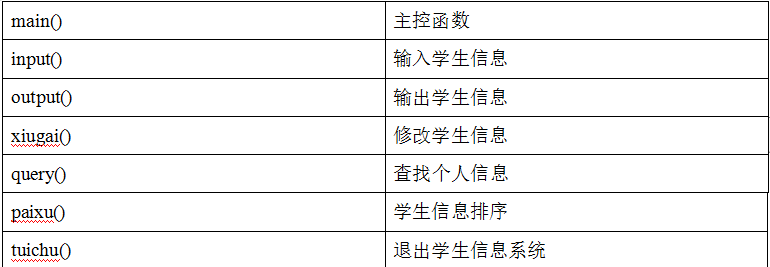3.6处理流程

（1）学生成绩管理系统主界面模块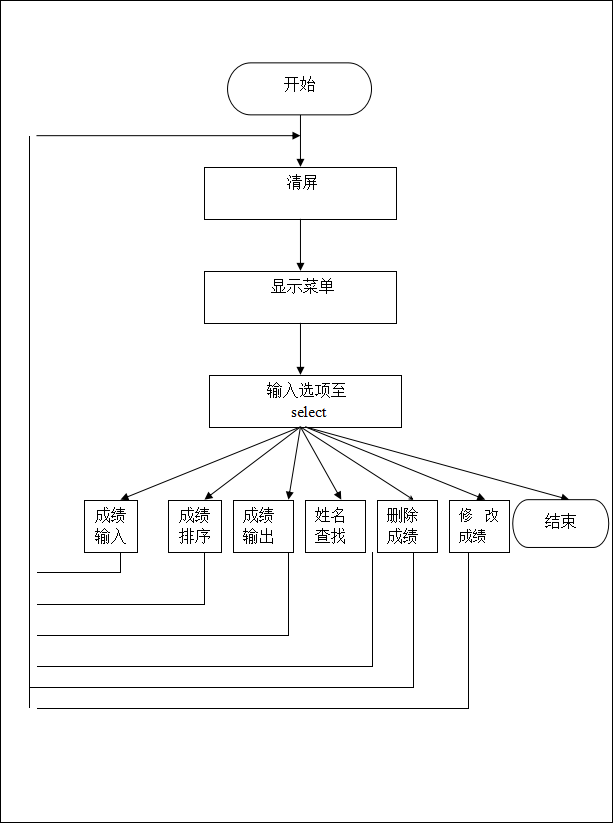（2）成绩输入模块 和（3）成绩排序模块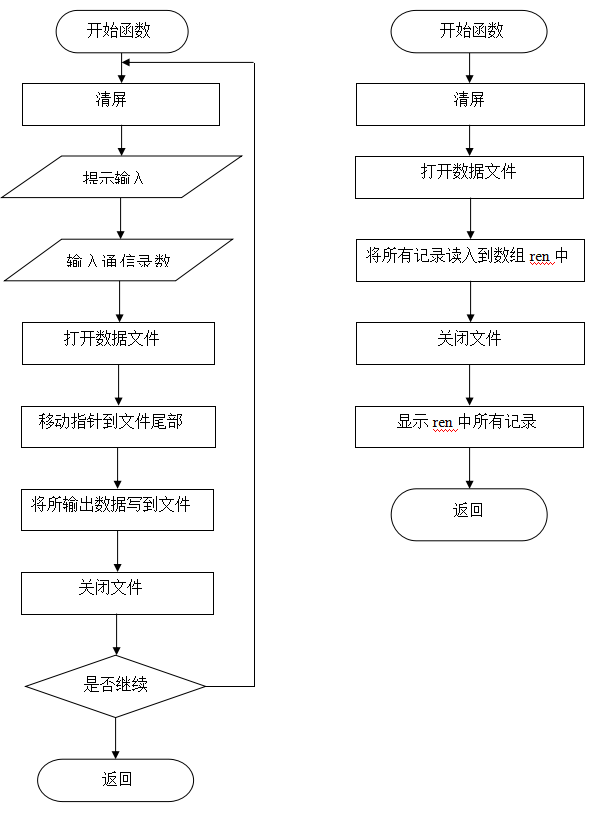（4）删除记录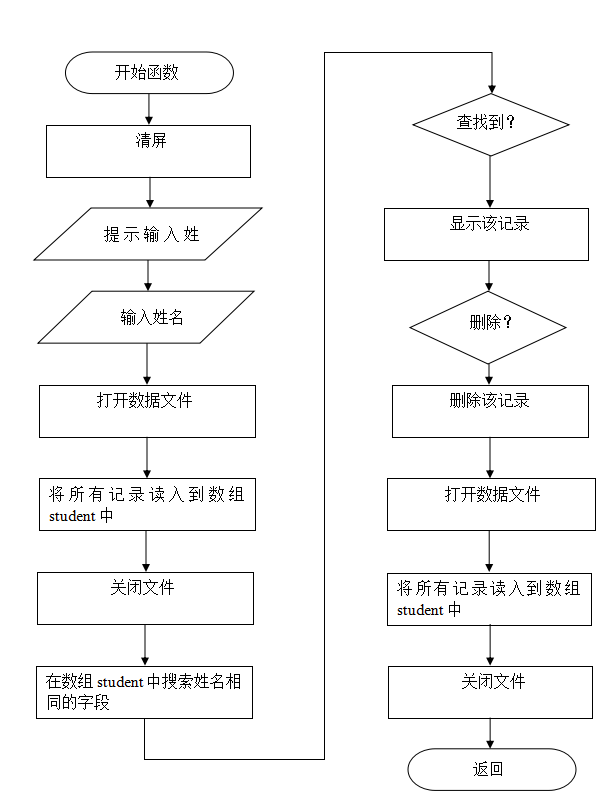（5）修改记录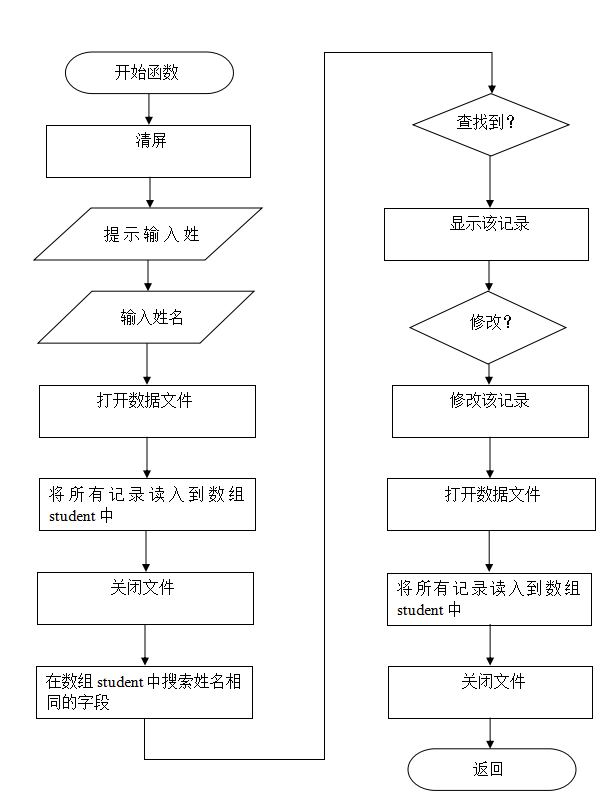（6）查找信息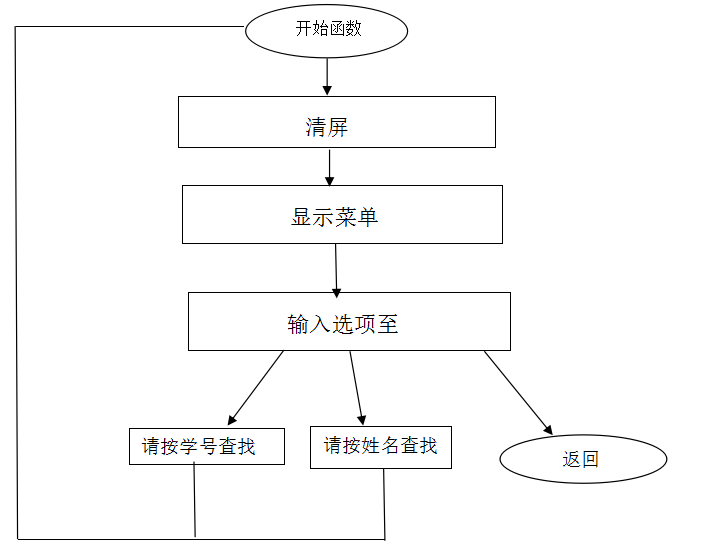四、结论

学生成绩管理系统的编写，看似杂乱无头绪，实际上却并不如此。它的每一个功能都能分成模块，有句话说的好，大事化小，小事化了。C语言编程也正式如此，把一个大的程序分为若干相互独立的模块，这样使完成每一个模块的工作变得单纯而明确，这样不仅降低了难度还使得思路清晰，很容易编写出要做的程序，从而为设计一些较大的软件打下了良好的基础。

分析问题：

学生成绩管理系统的整体功能都包括、增加成员、删除成员、查看成员、修改成员、登陆口令五大板块，而查看有包括学号，姓名，大学语文成绩，大学数学成绩，计算机成绩，总成绩，平均分七个模块。

设计的数据对象包括整形：学号。字符型：姓名、3门课程成绩、总成绩、平均成绩。
数据结构线性数据结构，定义人数上限为1000.
算法的设计：

自顶向下
现有全局，在进行整体设计，然后再进行下层的设计，逐步实现精细化。采用这种方法能够做到胸有全局，能全盘考虑，不至于顾此失彼，头重脚轻。

逐步细化
一步步地将上层的任务分解成较小的、易于实现的任务，知道可以很简单实现为止。

编写源程序：

不求速度，但求质量！一步一个脚印，编写程序时候宁可速度慢些也要尽量保证质量，做到尽量不出错。
精力集中，全神贯注！写程序时候最忌讳三心二意，这样才能思路清晰保证质量。
保持一个良好的编程心态，不要被长长的代码吓到
程序的编译与运行：

查找错误是一件让人闹心的事，但是要注重技巧，注意平时经验的积累。
程序编译成功不要满足现状，要积极测试程序的可用性，对功能不理想，存在缺点的地方，积极的改进，完善。
五、结束语

在实训过程中，使我良好的锻炼了自己，首先是兴趣方面，通过编写通讯录管理系统使我更加喜欢编程，使我从以前的迷茫状态中解脱出来，使我了解了编程的真正意义。我想这对我以后的人生有着很大的帮助。其次通过编写通讯录管理系统使我对写程序有了一定的思路，不像以前无从下手，使我理解了算法的意义，使我懂得了模块化思想的好处。

通过一个星期的编写代码，使我对书本上的知识有了更深的理解，甚至有很多不懂的问题在这过程中自然而然的理解了。对期末考试更有了信心。在朱老师的悉心指导下，我对C语言有了更深刻的理解，对程序的算法，数据的定义，程序的改错等方面的能力有了很大提高，自身的注意力，耐心，和对编程的兴趣有了很大增长，使我获益匪浅。

六、参考书目：

谭浩强，《C语言程序设计》，清华大学出版社

附完整代码：

#include<stdio.h>
#include<string.h>    //比较字符串头文件//
#include<stdlib.h>   //实时检测总数头文件//
#define N 1000       //控制最大输入//
int n=0;             //实时检测的总数n//

/*定义结构体students*/
struct students
{
int num;          //学号-整型//
char name;     //姓名-字符型//
char chinese;     //大学语文-字符型//
char math;        //大学数学-字符型//
char computer;    //计算机-字符型//
char sum;         //总成绩-字符型//
char ave;        //平均分-字符型//
}student[N],student1;     //student[N]是结构体数组student1是结构体的初始化//

/*实时检测总数n*/
void count()
{
FILE *fp;         //定义一个文件指针fp//
fp=fopen("students.txt","at+");     //以“追加”方式打开students文本文件//
if(fp==NULL)                        //判断文件是否为空//
printf("Cannot open file!");
struct students stu1;
n=0;         //初始化//
while(1)
{
if(feof(fp))  //检测文件是否结束//
break;
n++;    //读取文件数//
}
n--;           //关闭文件数//
fclose(fp);    //关闭文件//
}

/*菜单*/
void main()
{
int i;        //获取用户在菜单中的选择//
while(i!=0)
{
system("cls");     //清屏//
printf("\t\t欢迎进入学生信息管理系统\n");
printf("\t\t==============================\n");
printf("\t\t*请择操作                    *\n");
printf("\t\t*1输入学生信息               *\n");
printf("\t\t*2输出学生信息               *\n");
printf("\t\t*3修改学生信息               *\n");
printf("\t\t*4查询学生信息               *\n");
printf("\t\t*5排序学生信息               *\n");
printf("\t\t*6退出                       *\n");
printf("\t\t==============================\n");
scanf("%d",&i);
system("cls");     //清屏//
count();     //实时检测总数n//
switch(i)
{
case 1:input();break;    //输入学生信息//
case 2:output();break; 	//输出学生信息//
case 3:xiugai();output();break;    //修改学生信息//
case 4:query();break;    //查询学生信息*/
case 5:paixu();output();break;    //成绩排序//
default:
printf("输入有错\n");     //错误纠正//
}
}
if(i==0)
tuichu();        //退出系统//
}

/*成绩输入*/
void input()
{
int i=0;     //学生人数初始化//
char c;
FILE *fp;     //定义一个文件指针fp//
count();     //实时检测总数n//
fp=fopen("students.txt","at+");    //以“追加”方式打开students文本文件//
do
{
printf("请输入学生的个人信息:\n");
printf("\n请输入第%d学生的信息:\n",n+1);
printf("请输入学生的学号:");
scanf("%d",&student[i].num);
printf("请输入学生的姓名:");
scanf("%s",student[i].name);getchar();
printf("请输入学生的大学语文成绩");
scanf("%s",student[i].chinese);
printf("请输入学生的大学数学成绩:");
scanf("%d",&student[i].math);
printf("请输入学生的计算机成绩:");
scanf("%s",student[i].computer);
getchar();
student[i].sum=student[i].chinese+student[i].math+student[i].computer;
student[i].ave=student[i].sum/3;
fwrite(&student[i],sizeof(struct students),1,fp);  //以方式将其写入students文本文件//
i++;
n++;
printf("\n\n\t\t\t\t是否继续输入(Y/N):");getchar();     //提示是否继续，从键盘
获得一个字符//
scanf("%c",&c);
}
while(c=='y'||c=='Y');
fclose(fp);
}

/*成绩输出*/
void output()
{
int i;
char c;
FILE *fp;      //定义一个文件指针fp//
fp=fopen("students.txt","rt");    //以“读取的” 方式打开students文本文件//
system("cls");     //清屏//
getchar();

printf("||------||-----||-----||-----||------||-------||-------||------||");
printf("|#学号-----姓名------大学语文------大学数学------计算机------总成绩
-----平均分---#|\n");
do{
for(i=0;i<n;i++)
{
printf("%d",student[i].num);
printf(" %d",student[i].name);
printf("%d",student[i].chinese);
printf(" %d ",student[i].math);
printf("%d ",student[i].computer);
printf("%d",student[i].sum);
printf(" %d ",student[i].ave);

}
printf("|------||-----||------||-----||-----||-----||-----||------ |");
printf("\n\n\t\t\t\t是否继续其它操作(Y/N):");     //提示是否继续//
scanf("%c",&c);
c=getchar();
}while(c=='y'||c=='Y');
fclose(fp);      //关闭文件//
}

/*成绩修改*/
void xiugai()  {
int i,tp,num1;
char c;
FILE *fp;     //定义一个文件指针fp//

lb:
printf("\n按学号修改\n\n");
printf("请输入要修改的学生学号\n");
scanf("%d",&num1);

for(i=0;i<n;i++)
{
if(num1==student[i].num)
{
printf("要修改的学生信息为：\n");
printf("学号\t姓名\t大学语文成绩\t大学数学成绩\t计算机成绩\t总成绩\t
平均分\n");
printf(" %d ",student[i].num);
printf(" %d ",student[i].name);
printf(" %d ",student[i].chinese);
printf(" %d ",student[i].math);
printf(" %d ",student[i].computer);
printf(" %d ",student[i].sum);
printf(" %d ",student[i].ave);
do {
printf("请选择要修改项：\n");
printf("1学号\n2姓名\n3大学语文成绩\n4大学数学成绩\n5总成绩\n6平
均分\n");
scanf("%d",&tp);
switch(tp)
{
case 1:
{	 printf("请输入学号:\n");
scanf("%d",&student[i].num);
goto la;
}
case 2:
{ printf("请输入姓名:\n");
scanf("%s",student[i].name);
goto la;
}
case 3:
{ printf("请输入大学语文成绩:\n");
getchar();
scanf("%c",&student[i].chinese);
goto la;
}
case 4:
{ printf("请输入大学数学成绩:\n");
scanf("%d",&student[i].math);
goto la;
}
case 5:
{printf("请输入计算机成绩:\n");
scanf("%s",student[i].computer);
goto la;
}
case 6:
{ printf("请输入总成绩:\n");
scanf("%s",student[i].sum);
goto la;
}
case 7:
{ printf("请输入平均分:\n");
scanf("%s",student[i].ave);
goto la;
}
}
}while (tp<8);
la:	  printf("是否继续修改(y/n)\n");     //提示是否继续//
getchar();
scanf("%c",&c);
if(c=='y'||c=='Y')  goto lb;
}
}
fp=fopen("students.txt","w+");   //以“读写” 方式打开students文本文件//
for(i=0; i<n;i++)
fwrite(&student[i],sizeof(struct students),1,fp);  //以 方式将其写入students
文本文件//
fclose(fp);    //关闭文件//
}

/*成绩查询*/
void query()
{ int querymode;     //子菜单选择//
int i,j=0,findnum;
char findname;
int findok,t=0;     //定义一个判断标志的整型变量findok//
char findct;        //定义一个用于判断是否继续查找的字符变量findct//

FILE *fp;         //定义一个文件指针fp//
count();     //实时检测总数n//
fp=fopen("students.txt","r");       //以“只读” 方式打开students文本文件//
system("cls");     //清屏//

/*查询菜单*/
printf("\t\t信息查询");
printf("\n\t1按姓名查询");
printf("\n\t2按学号查询");
printf("\n\t0返回上级菜单");
printf("\n请输入您的操作:");
scanf("%d",&querymode);

/*查询操作*/
switch(querymode)
{case 1:
{     printf("请输入要查询学生的姓名:\n");
getchar();
scanf("%s",findname);
for(i=0;i<N;i++)
{
文本文件//
if(strcmp(student[i].name,findname)==0)        //比较字符串//
{
j++;
printf("\n查寻到的学生为:\n");
if(j==1)
、
printf("|------||-----||------||------||------||-------||------||-----|");
//仅在第一次显示//
printf("|#学号-----姓名------大学语文------大学数学------计算机------总成绩-
----平均分---#|\n");

printf("%4d%d%d%d%d%d%d%d",student[i].num,student[i].name,
student[i].chinese,student[i].math,student[i].sum,student[i].ave);
}

}
if(j==0)
printf("\n>>>查无此人！");

//提示是否继续//
printf ("\n是否继续查找(Y/N)?");
getchar();
getchar();}break;

case 2:{ printf("请输入要查询学生的学号:");
scanf("%d",&findnum);
for(i=0;i<n;i++)
{
//以结构体students的方式从文件fp中读取,将其读入students文本文件,每次读入一个
学生的信息//
if(student[i].num==findnum)      //整型数字的比较//
{findok=1;break;}
else findok=0;
}
if(!findok)
printf("\n>>>查无此人！");
else
{ printf("\n查寻到的学生为:\n") ;
if(j==1)
printf("|-------||------||------||-------||-------||-------||-------||------|");
//仅在第一次显示//
printf("|学号-----姓名------大学语文------大学数学------计算机------总成绩-----平均分---|\n");
printf("%4d%d%d%d%d%d%d%d",student[i].num,student[i].name,
student[i].chinese,student[i].math,student[i].sum,student[i].ave);
}
//提示是否继续//
printf ("\n是否继续查找(Y/N)?");
getchar();
findct=getchar();     //从键盘输入一个判断字符，并将它赋值给字符变量
findct,用于以下的if语句的判断 //
if(findct=='Y' || findct=='y')
query();
}
}
fclose(fp);    //关闭文件//
}

/*成绩排序*/
void paixu()
{
int i, j;
FILE *fp;       //定义一个文件指针fp//
system("cls");      //清屏//
count();       //实时检测总数n//
for(i=0;i<n-1;i++)
for(j=0;j<n-1-i;j++)    //按学号对学生信息进行排序//
{
if(student[j].num>student[j+1].num)   //整型数字的比较//
{
student1=student[j];
student[j]=student[j+1];
student[j+1]=student1;
}
}
fp=fopen("students.txt","w+");  //以 “读写”方式打开students文本文件//
for(i=0;i<n;i++)
fwrite(&student[i],sizeof(struct students),1,fp);  //以结构体students的方式从
文件fp中读取，将其写入students文本文件，每次写入一个学生的信息//
fclose(fp);      //关闭文件//
}

/*退出系统*/
void tuichu()
{
char c;
system("cls");      //清屏//
printf("\n\n\t\t谢谢使用\n\n\n");
printf("\n\n\t\t制作人:李姣 渠莉 宝珠 朱佳 刘妍\n");
getchar();
c=getchar();       //取用户输入，回显//
}

需要实训报告的同学，请在下方留言，看到会一个个回复的。

或者直接扫描下方公号，发送 成绩系统 三个字，获取下载源码。如果失效了，再请直接私信或留言，欢迎大家一起探讨学习。

相关文章推荐：

1、 C语言学生成绩管理系统源代码 ★★★★★

2、 C语言学籍管理系统源代码 ★★

3、C语言学生成绩管理系统设计 《C语言程序设计》实训报告 ★★★

展开全文学生成绩管理系统 成绩管理系统 管理系统
• 文章目录20201015-成信大-C语言程序设计-20201学期《C语言程序设计B》C-trainingExercises02成信大-C语言程序设计上机作业-参考**20201学期《C语言程序设计B》C-trainingExercises02**P134P710P733P259P711 ...
• 文章目录20201022-成信大-C语言程序设计-20201学期《C语言程序设计B》C-trainingExercises11P211P212P213P214P731P830P215P745 20201022-成信大-C语言程序设计-20201学期《C语言程序设计B》C-trainingExercises11 ...
• 文章目录20201022-成信大-C语言程序设计-20201学期《C语言程序设计B》C-trainingExercises10P125P127P232P753P716P724 20201022-成信大-C语言程序设计-20201学期《C语言程序设计B》C-trainingExercises10 P125 /* ...
• 谭浩强C语言程序设计第五版 第4章 课后习题 答案 1.什么是算术运算？什么是关系运算？什么是逻辑运算？ 答：算术运算时数学里基础的加减乘数求余数等；关系运算时求两个或者多个变量或者表达式之间的关系；逻辑运算...
• 1.判断输入字符种类 #include #include int main() { char c; printf("plz enter character:\n "); c=getchar(); if(c){ printf("\n the charater is a control charater");... }else if(c>='0'&设计
• ## C语言程序设计视频

千次阅读 热门讨论 2018-10-14 11:04:11
C语言程序设计的明码：【苏小红】 09461268081145416196 下载链接的解压码见评论区。
• C语言是非常流行的高级编程语言之一，是很多同学的入门编程语言，是建立编程思想的基础课程。 本视频课程是配合学生在学习了基本的理论课程的同时，进行编程实践，帮助初学者有效的进行上机实践，视频主要通过实例...c语言 c c语言链表 c语言指针
• ## 大一C语言程序设计练习题(四)

千次阅读 多人点赞 2020-12-13 21:59:00算法
• ## C语言程序设计

万次阅读 2009-12-14 19:13:00
C语言程序设计》第三版 潭浩强(娃娃书入门) 《C程序设计语言（第2版·新版）》 英文名字《The C Programming Language》 本书是由C语言的设计者Brian W. Kernighan和Dennis M. Ritchie编写的一部介绍标准C语言及其...语言 c 数据结构 standards
• C语言程序设计知识点总结我与C语言的故事第二章C数据类型C Hello World 实例C语言基本语法分号 ;注释标识符关键字C 中的空格数据类型基本类型书写整数小数字符型常量字符串常量C 变量C 常量整数常量浮点常量字符常量...字符串 编译器
• 最近忙于学习C语言程序设计，考计二C。都搞得我头晕眼花，天天都是刷题。马上就要考计二C了。我想总结一下我对C语言的理解。 C不同于java的最大区别在于C是面向过程，而java是面向对象。刚开始我也搞不清到底区别在...java
• ## C语言课程设计报告-菜单设计

万次阅读 多人点赞 2019-07-02 18:55:03
源代码：https://blog.csdn.net/queen00000/article/details/94468911 xxx大学 C语言课程设计报告 ...
• ## C语言程序设计专栏索引

千次阅读 多人点赞 2021-01-24 01:21:16
C语言编程＞第一周 ② 输入圆的半径r的值，输出其面积。 C语言编程＞第一周 ③ 输入某年某月某日，判断这一天是这一年的第几天。 C语言编程＞第一周 ④ 输出9*9乘法表。 C语言编程＞第一周 ⑤ 有1、2、3、4，4个数字...
• C语言程序设计》专升本考试大纲 一、总要求 本课程地位：本课程是计算机专业的核心课程之一。 本课程性质：本课程是计算机专业的专业基础课程 本课程了解、熟悉、掌握的主要内容：了解C语言的主要特征、常用算法...专升本
• 单片机C语言程序设计实训100例大集合 本书基于Keil μVision程序设计平台和Proteus硬件仿真平台，精心编写了100余个8051单片机C语言程序设计案例。完整的资料已经上传到闯客网技术论坛了，关于这个资料已经创建了一...单片机 嵌入式
• 该课程为“C语言程序设计”系列课程中的第三部“进阶篇”。作为终结篇C语言教程，介绍了在实际应用中应用广泛的结构体数据表示和处理、利用文件进行输入输出、利用多文件组织项目开发，并结合对程序设计的进一步...c语言 c c语言链表 c语言指针
• C语言程序设计第五版 谭浩强 第六章 答案 1.用筛选法求100以内的素数。 #include <stdio.h> #include <math.h> void main() { int i,j,a,b; for (i=1;i<=100;i++) { a[i]=i+1; for ...
• 本人决定将搜集的一些C语言程序设计经典书籍拿出来跟朋友们分享，需要的朋友请直接下载。 下载地址链接: https://pan.baidu.com/s/1SC74Qg-SXyGJMt-To5NrOA 密码: uep8 1.Kernighan、Ritchie，《C程序设计语言......

# c语言程序设计报告c语言 订阅